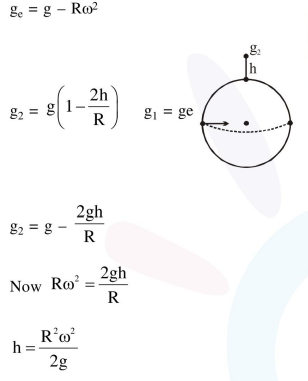# The acceleration due to gravity on the earth's surface

Question:

The acceleration due to gravity on the earth's surface at the poles is $\mathrm{g}$ and angular velocity of the earth about the axis passing through the pole is $\omega$. An object is weighed at the equator and at a height $\mathrm{h}$ above the poles by using a spring balance. If the weights are found to be same, then $h$ is : $(h< 1.$\frac{\mathrm{R}^{2} \omega^{2}}{8 \mathrm{~g}}$2.$\frac{\mathrm{R}^{2} \omega^{2}}{4 \mathrm{~g}}$3.$\frac{\mathrm{R}^{2} \omega^{2}}{\mathrm{~g}}$4.$\frac{R^{2} \omega^{2}}{2 g}\$

Correct Option: , 4

Solution: